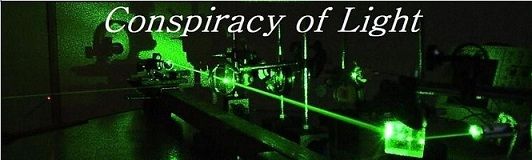The search for  new physics.

The Sagnac Effect: Does it Contradict Relativity?

By Doug Marett 2012

A number of authors have suggested that the Sagnac effect contradicts the original postulates of Special Relativity, since the postulate of the constancy of the speed of light is violated in rotating systems. [1,2,3] Sagnac himself designed the experiment of 1913 to prove the existence of the aether . Other authors have attempted to explain the effect within the theoretical framework of relativity, even going as far as calling the effect “relativistic”. However, we seek in this paper to show how the Sagnac effect contravenes in principle the concept of the relativity of time and motion.  To understand how this happens and its wider implications, it is necessary to look first at the concept of absolute vs. relative motion, what the Sagnac effect implies about these motions, and what follows logically about the broader notions of space and time.

Early Ideas Regarding Rotation and Absolute Motion:

The fundamental distinction between translational and rotational motion was first pointed out by Sir Isaac Newton, and emphasized later by the work of Ernest Mach and Heinrich Hertz.  One of the first experiments on the subject was Newton’s bucket experiment of 1689. It was an experiment to demonstrate that true rotational motion cannot be defined as relative rotation of a body with respect to surrounding bodies – that true motion and rest should be defined relative to absolute space instead.   In Newton’s experiment, a bucket is filled with water and hung by a rope. If the rope is twisted around and around until it is tight and then released, the bucket begins to spin rapidly, not only with respect to the observers watching it, but also with respect to the water in the bucket, which at first doesn’t move and remains flat on its surface.Eventually, as the bucket continues to spin, the water starts to rotate as well, as can be seen from the concave shape of its surface. This concaving of the water shows that it is rotating, even though it is now at rest with respect to the co-rotating bucket. Newton pointed out it is not the relative motion of the bucket and water that causes the concavity of the water. The concaving of the water suggests that it is rotating with respect to something else, far more remote.  In Newton’s thinking, this showed rotation relative to say…absolute space. This is contrary to the idea that motions can only be relative.

Now an observer who is sitting in the bucket and thereby rotating with it can see that the water is concave but may not be able to see or feel that he is rotating with respect to the surroundings, so the cause of the concavity may not be obvious, particularly if the rotation is slow and his view of the surroundings is blocked. On the other hand, an observer who is standing near the bucket (stationary) will see a concave shape of the water’s surface that is consistent with his observation of the bucket rotating in front of him. So although both observers see the effect of rotation (concave surface) they cannot necessarily agree on the cause.

It is sometimes argued that because a stationary frame can be identified (the observer near the bucket), there is no reason to ask “rotating with respect to what?” However, we wish to show that although multiple stationary frames can be identified depending on the circumstances, there is only one truly unique stationary frame, and this is the frame of absolute space, a.k.a “the fixed stars.”

The Sagnac Effect:

While originally these ideas were applied to mechanical effects, with the Sagnac effect it becomes clear that the same principles apply to light, and the frame of reference in which light speed can be shown (or not shown) to be constant. Consider the Sagnac effect in the context of Newton’s bucket experiment. Instead of a rotating bucket, we have a rotating disk, and on the rim of the disk are two light paths originating from a source, one going clockwise and the other going counter-clockwise around the rim of the disk, and returning to a detector where their time of flight is measured. Since the transit time is usually very small, the differences in the return times are determined by a phase difference between the two returning beams. For the Sagnac effect, we call this Case 1. The speed of light (C) in the stationary frame is assumed to be C ~= 3 x 108 m/s.

If we now rotate the disk clockwise at velocity v, we find that the blue beam arrives back at the detector before the red beam – in fact, the difference in  the  velocity  of  light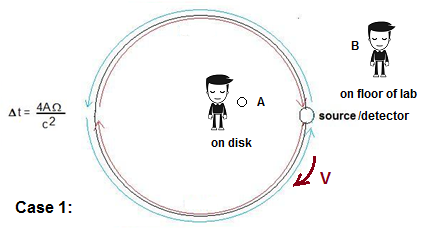turns out to be 2*v, because the blue beam travels at C+v and the red beam at C-v.  Just like the concave water, all observers agree that the blue beam has arrived before the red beam, but again, like in the case of Newton’s bucket, the explanation of why depends on the perspective of the observer.  For the observer B it appears that the detector has approached the oncoming blue beam and has receded from the red beam, and it is the path length change with respect to the two beams that accounts for the apparent difference in the speed of light. On the other hand, the observer at A who is rotating with the disk has observed that the source and detector are stationary from his perspective, as are the paths, so he can only conclude (assuming no foreknowledge that he is rotating) that the light has actually travelled at two different velocities around the disk, being C+v and C-v.  Who is right? Well, the problem is this – from the point of view of special relativity, there are no preferred frames of reference, there are only relative frames of reference, so we can’t argue that frame B is better than frame A without defeating the underlying premise of the theory. In special relativity (SR), the position of the observer is supposed to be arbitrary. However, if we accept that perspective A is legitimate, we defeat the first postulate of SR, namely:

“that light is always propagated in empty space with a definite velocity c which is independent of the state of motion of the emitting body”. – Einstein 

Further, on the relativistic notion that ”it is impossible to detect motion by measuring differences in the speed of light”, as had seemingly been proven by the Michelson-Morley experiment, the Sagnac experiment shows that this can in fact be done. Also, observer A can determine that it is he who is rotating, and not his surroundings.

Now to address the idea of stationary states we need to ask the following question: Is there a single unique observer (frame of reference) for which the speed of light is always constant in a Sagnac interferometer? In fact, the speed of light measured by observer B is not exactly constant either, a conundrum which we will explain below.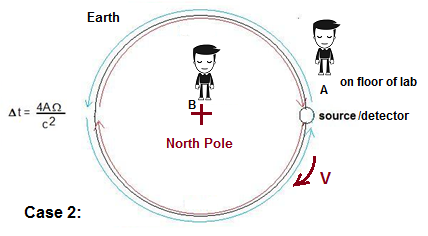In case 1 we recall that observer B in the lab was stationary and noted that the speed of light should be approximately C in both directions. Let’s expand our interferometer now to encompass the earth. We move our observer B to the North Pole, where he hovers, not rotating, and our observer A is placed in the lab on the earth’s surface, along with our source and detector. Our circular light path is now the equator of the earth. This configuration is in fact the experiment first proposed by Albert Michelson in 1904. Since the source, detector, and observer A are all on the surface of the earth, from their perspective they are stationary (excluding fore-knowledge). However, as before, A observes the speed of light reaching the detector after traveling around the globe to be C+v and C-v for the blue and red light paths, respectively, where v is the rotational velocity of the earth on its axis (465 m/s). Also, observer B at the North Pole can see the Earth rotating under him, so from his perspective the paths are again unequal and the speed of light is about constant from his perspective.

So now we can see that the lab frame is not a unique perspective – in case 1 the speed of light was approximately constant in the lab frame, in case 2 it is variable. However, even in case 1, the so called “stationary observer” in the lab will be able to detect the slow rotation of the much smaller lab-sized ring due to the earth spinning on its axis, of up to 15 degrees per hour, so the speed of light measured by this observer will not be constant either, but will differ by the much smaller amount C+/- (r * W) where r is the radius of the loop and W is the angular velocity of rotation at the observer’s latitude.

However, the North Pole could be construed as an approximately stationary perspective for both case 1 and 2. So is the speed of light always constant with respect to the non-rotating Earth (the so called ECI frame used in GPS)? Again, we can show this not to be true.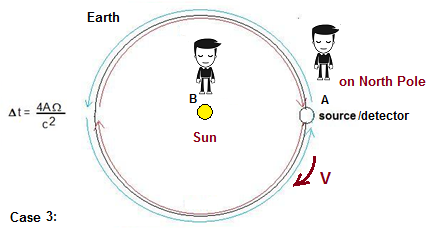If we consider a third scenario (Case 3), where B is on the Sun and A is on the North Pole, and the light paths go around the orbit of the Earth, we find that now the North Pole perspective sees a variable speed for C (+/- the orbital velocity of the earth which is ~30 km/s) and only the observer on the sun sees an approximately constant C based on his observation that the two light beams have followed longer or shorter paths. But using the same reasoning as in case 2, the observer B on the sun will not measure exactly C either, but C+/- a much smaller value, again being C+/- (r * W) where r is the radius of the galaxy to our sun and W is the angular velocity of our sun around the galaxy.

This line of reasoning can continue to be expanded to larger and larger scales – for example, if A is standing on the Sun and B is at the center of the galaxy, then the center of the galaxy becomes the (approximate) preferred perspective. In fact, the only way that we can define an observer for which all circumstances the speed of light C is exactly constant in a circular path, is to place the observer B stationary with respect to the universe.Any light following a circular path with respect to B will always be observed to be exactly C, but only from this perspective. For all other velocity frames, the speed of light can be variable. In reaching this conclusion, we have established that the Sagnac interferometer implies that there must exist only one unique frame of reference for rotation, and this is the universe itself – absolute space, a.k.a. “the fixed stars”.  So to answer the question “with respect to what does the Sagnac interferometer rotate?” we must answer, “absolute space, the fixed stars.” But in doing so, we are compelled to reject the idea of the relativity of motion that is the cornerstone of Einstein’s theory.

Relativists claim that the Sagnac effect can be accounted for by assuming a constant light speed on the rotating disk and by factoring in the time dilation of the rotating observer. This uses a backwards mathematical transformation from the stationary observer. This mathematical treatment, attributable to Langevin and repeated for example by E.J. Post, was decisively disproved by the Dufour and Prunier experiments. See Appendix A for the full discussion.

______________________________________________________________________________________________

GPS and the Sagnac Effect – GPS satellites must use clocks synchronized to the Earth Centered Inertial frame (ECI) – i.e. their satellite observer clock coincides with the non-rotating observer B on the North Pole of Case 2. If they use clocks synchronized to the observer A on the earth’s surface in the Earth Centered Earth Fixed frame (ECEF) of case 2 then the speed of light between the satellite and earth is no longer measured to be C, introducing a propagation range error and thereby an error in the receivers estimated position. This procedure has been used in practice now for decades.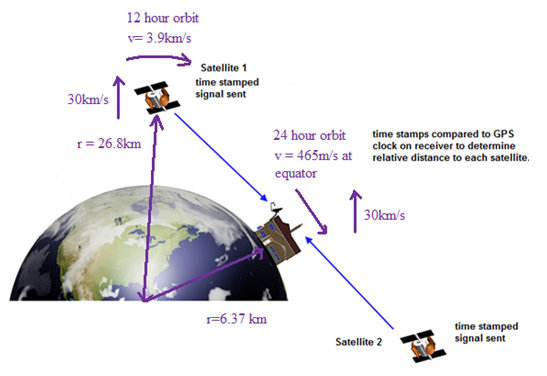______________________________________________________________________________________________________________________

The Non-Relativity of Time

The Sagnac effect also does for time what it does for light. Many of us know Einstein’s famous twins paradox, where one twin stays on earth while the other travels in a rocket at near light speed, returning years later to meet his twin. When they meet again, the travelled twin is younger than the stay at home twin, because according to Einstein, the high velocity of the second twin meant that time progressed slower for him than the stay at home twin.

This thought experiment was criticized by Dingle, who aptly pointed out that if Einstein argues that a moving clock counts slower than a stationary one, but also presumes all motion to be relative, then we should be able to argue with equal justification that it is the earthbound twin who has moved away at velocity from the rocket bound twin, and that the former’s clock has counted slower instead.

An attempt to resolve this issue was the Hafele and Keating Experiment. Hafele and Keating [9,10] performed an experiment in 1971 to test Einstein's predictions regarding the dilation of time in clocks moved at some velocity with respect to a stationary clock. In the opening statement of the first of two papers on the subject, the authors refer to the debate surrounding the "twins paradox" and how an experiment with macroscopic clocks might provide an empirical resolution. In Hafele and Keating's experiment, they flew cesium clocks around the world in opposite directions near the equator, and then measured how much time they have gained or lost when they return to the start point as compared to a stationary cesium clock to which they were originally synchronized. The experiment of Hafele and Keating (HK) actually differs from the twins paradox in some important ways - firstly, in the paradox, the traveling twin moves in a straight line to a distant point then turns around and comes back, with a series of accelerations and decelerations. In the HK experiment, the journey is circular and the clocks never leave the earth’s influence. In following a circular path, the HK experiment becomes a Sagnac effect experiment where it is clocks that are moving rather than beams of light.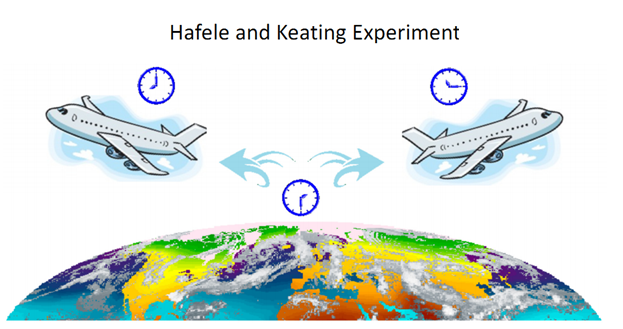From the perspective of the observer on the ground, the moving clocks of Hafele and Keating have experienced similar motions with respect to the stationary earth bound clock. If their motions were truly "relative", then by the rules of SR both should arrive back at the starting point having experienced the same amount of time dilation in the same direction.    The actual result was that one travelled clock increased in its time rate and the other decreased. This is the identical result to what would be expected for light in the Sagnac experiment Case 2, where one beam travels faster than expected, and the other slower than expected. And as before, it implies that the only observer who sees all clocks count at a rate proportional to their velocity is the observer at the North Pole, who is in the non-rotating, geocentric (ECI) frame of the earth.

As Murray pointed out, Hafele and Keating interpreted their result by following a revised form of relativity attributable to Builder, who they reference in their first paper in 1971. In Builder's "Ether and Relativity" he summarizes his view as follows :

"The relative retardation of clocks, predicted by the restricted theory of relativity, demands our recognition of the causal significance of absolute velocities. This demand is also implied by the relativistic equations of electrodynamics and even by the formulation of the restricted theory itself. The observable effects of absolute accelerations and of absolute velocities must be ascribed to interaction of bodies and physical systems with some absolute inertial system. We have no alternative but to identify this absolute system with the universe. Thus in the context of physics, absolute motion must be understood to mean motion relative to the universe, and any wider or more abstract interpretation of the "absolute" must be denied. Interactions of bodies and physical systems with the universe cannot be described in terms of Mach's hypothesis, since this is untenable. There is therefore no alternative to the ether hypothesis. "

Builder’s conclusion that there must be "an absolute inertial system" re-introduces the preferred frame of Lorentz back into relativity, the very thing that Einstein sought to eliminate.

If we are to accept Builder's hypothesis of a universal preferred frame for motion (ether), then we are compelled to accept the other critical tenet of Lorentz over Einstein, that it is the speed of light, and not time, which is the fundamental variable in our universe. In accepting a notion of absolute motion, or a preferred frame, we are as Dingle says, rejecting the notion of "relative motion". Despite Einstein borrowing heavily from Lorentz's equations and theory, and the fact that both theories predict similar results for the same experiments, the two theories are philosophically antithetical - and as such the conclusions about the nature of space and time result in entirely different models of the universe.

Let’s look at the implications of the Hafele and Keating Experiment on the relativity of time. Similar to our original case 3, we expand the travelling clock experiment to the orbit of the earth. Rather than planes, we put cesium clocks on two rockets and have them take off from the surface of the earth at equal escape velocities (say 12 km/s), but set to travel in opposite directions around the sun before returning to the earth, as in the figure below. The rockets maintain the same velocity with respect to the earth’s surface throughout their voyage. Both rockets arrive back on the earth at around the same time, and again at the same velocity with respect to the earth, at 12 km/s.  As expected, the rocket on the left going counter-clockwise arrives with its clock rate slowed with respect to the earth clock, and the rocket on the right travelling clockwise has had its clock rate sped up with respect to the earth clock, even though their velocity with respect to the earth’s surface clock was the same. From the perspective of the earth (assuming no foreknowledge that they are  rotating),  the  path  lengths  for  each  rocketappear to be the same. However, an observer B on the sun would see that the rockets had to travel at different speeds with respect to the sun in order to be travelling at the same speed with respect to the orbiting earth. The paths that they travelled would also appear to be different lengths when viewed from the sun. Only the observer on the sun would agree that the SR postulate that a clock decreases it rate with velocity is found to be (approximately) true. The earth observer A sees this to be false – for him, the clocks have travelled identical paths at identical speeds, yet the clocks have gone out of synchronization.  This invalidates the notion that the ECI frame of the earth is a unique frame of reference for rotation with which the laws of SR hold true.

Again, we can extrapolate this thought experiment to the edge of the galaxy, and further to the entire universe.  For clocks rotating around an observer, the only unique perspective where ultimately any rotational motion will always lead to a slowing of the clock rate is the stationary frame of the universe. In any other velocity frame, it will always be possible to create orbiting clocks that appear to speed up in rate with respect to our observer. This is the same conclusion as earlier for the speed of light, except now applied to time.

These rotation experiments have implied that there is a an absolute space with which we can define a reference speed of light C, and a preferred frame of reference for a time clock for which all other moving clocks will count at a slower rate.

Since Einstein invented the idea of a variable time in order to explain the constancy of the speed of light, should it not follow then that if we have shown that the speed of light is not always constant for moving observers, then perhaps instead it is “time” that is constant, and the velocity of light is variable depending on our motion with respect to absolute space? Since translational experiments always show a constant measured speed of light, unlike the Sagnac experiment, perhaps apparent time dilation is our only indirect evidence that the speed of light is changed in a hidden way during translational motion. In order to prove this we would need to design a clock that doesn’t depend on the speed of light in its counting principle, and see if this clock is unaffected by time dilation. Again, we will turn to the Sagnac effect and rotation to design such a clock. But first, let’s examine the basis for time dilation in Einstein’s theory.

The Light Clock Analogy

When we see a supernova explode in the sky, we do not say “that event just happened”, since we know the light from that event has taken perhaps thousands of years to reach us from some distant point in space. We would say, rather, that the explosion happened thousands of years ago. This is because our judgement of the simultaneity of events is independent of the speed of light. Rather the speed of light might be used to insinuate when an event could have happened in the past, based on the propagation delay. So why then would we believe that the rate of time itself depends on the speed of light? Consider Einstein’s analogy, often reproduced, of a light beam bouncing between two mirrors. In his analogy, two horizontal mirrors face each other and one mirror is spaced above the other by a distance d. A light pulse bounces vertically between the two mirrors as shown on the left.  The time it takes for the pulse of light to do a round trip (from the top mirror to the bottom and back) is twice the distance d divided by the speed of light. This is his light clock.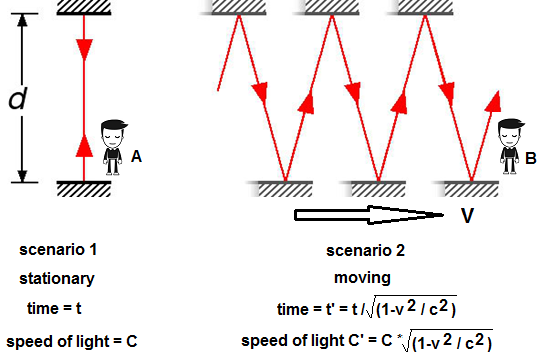Suppose the "light clock" were traveling sideways at a very high (but constant) speed of 0.5 C. Now the pulse would follow the "saw tooth" path shown on the right side of the drawing. Since light speed is the same as before (remember, the speed of light is not changed by the speed of its source), it will take longer to make a round trip. So our "light clock" takes longer to count out its intervals. Another way of saying this is that the clock "ticks" more slowly.

But here lies the problem. This analogy works because the speed of light is constant as seen by observer A. From his vantage point, he can see both clocks working, and although the speed of light is travelling at the same rate in scenario 2, it has taken a longer path to hit the moving mirrors. In taking a longer path, it has taken more time to reach the end (based on A’s clock – 1.1546 x longer or t/0.866 = t’). But because we have already said that the rate of time is independent of the speed of light, we cannot assume that “real” time has passed slower for observer B than observer A, we can only conclude that B’s clock has counted mechanically slower because it has taken longer for the light to travel the distance between scenario 2’s mirrors. Now, if observer B measures the speed of light in his moving frame using “real” time, i.e. a clock not affected by the speed of light, he should conclude that the speed of light is slower than C, namely 0.866*C = C’. This is exactly like the situation of the Sagnac interferometer – observer B is unaware that he is moving, so he perceives the mirrors to be opposite each other and separated by distance d, so he would measure the speed of light to be less than C. He is unaware that from the perspective of observer A, the path d has lengthened. So why does he conclude that the speed of light is instead C? This is because he measures the time elapsed using a clock that is based on the length of time it takes for light to pass between his own moving mirrors. In effect, his moving clock is calibrated by the speed of light seen in his own frame (which is 0.866*C), so consequently, his clock counts slower by exactly this amount (t/0.866). This makes him falsely measure that the light beam to have arrived at velocity C rather than C * 0.866.

If the speed of light was actually C in his frame, i.e. C = C’, then his moving light clock would count faster (now t = t’) then although he would still measure the speed of light to be C with his clock, the time rate difference between A and B would disappear! Since the slowing of clocks (apparent time dilation) does occur in reality, this implies, just like in the Sagnac experiment, that the speed of light is only actually C in the stationary frame, and in the moving frame it is actually C *  0.866, but is erroneously measured as being C in his frame due to the slowing error introduced into his moving clock by the longer light path.

We can go one step further – the light clock analogy explains why every translational experiment to measure the speed of light shows that the speed of light is always C – because the clocks used to measure C are calibrated to the speed of light in their frame, this built-in correction factor always adjusts the measured speed back to C. However, in every rotational experiment, it is trivial to measure a speed of light that is not C. This is because, as we showed with Hafale and Keating, clocks moving in rotating paths around a disk or sphere will go out of synchronization with each other – using a common origin as a reference and a circular path to the detector confounds the self-correcting ability of a light clock, and the underlying difference in the speed of light with respect to the origin is revealed.

The Sagnac Clock:

As we said earlier, in order to prove that time dilation is an illusion,  we would need to design a clock that does not depend on the speed of light in its counting principle, and see if this clock is unaffected by time dilation. Again, we turn to essential feature of the Sagnac effect, rotation, to design such a clock. This concept was first proposed in our article “The Paradox of the Clocks in the Canaries” in 2010.

The simplest time dilation effect to test is gravitational time dilation, the speeding up of clocks with altitude. According to Einstein, the rate of time follows the gravitational potential, so a clock on the surface of the earth should count more slowly than a clock at some high altitude, such as at the top of a mountain. Time is alleged to be moving into the future faster at the top of a mountain.  So we need a clock on the Earth that is unaffected by altitude. Let's say we use the rotation of the earth as our clock instead of a conventional cesium clock. After all, our human concept of time, and the units that compose it (days, hours, minutes, seconds) are already derived from the rotation period of the earth, so it should be the ideal clock for comparison. For each observer, we could use as our reference the position of the sun in the sky, or the position of a Foucault pendulum, or most preferably the position of the fixed stars in sidereal time. If we measure the sidereal day (23.93447 hours) as the time for one earth rotation relative to the vernal equinox, using a sighting star as a reference, we can then place an observer A at the top of a mountain, and another observer B at sea level. Preferably the mountain observer is exactly vertical over the sea level observer, and this vertical line passes through the center of the earth, as shown in the picture below. We use as our example the mountain Pico del Teide on Tenerife, with an altitude of 3718 meters. We give each observer A and B a cesium clock, and a sidereal clock.  The sidereal clocks mark a tick when the reference star is directly overhead each day. The cesium clocks mark a tick when 23.93477 hours has passed in their local time. We then compare the four clocks to determine if they are counting at the same rate.

What happens is that when the reference star passes over the red vertical line passing through observer A and B, both sidereal clocks have no choice but to trigger at the same moment, differing only in the light propagation time between A and B. Because this propagation time is a fixed amount, it does not affect the rate at which the two clocks count – therefore the count rate is exactly the same. However, since both cesium clocks experience gravitational time dilation, the cesium clock at observer A must count faster than the cesium clock at observer B. If we had pre-synchronized the two clocks at sea level, then the cesium clock at observer A will begin to go out of synchronization withthe other three clocks, and the amount of time gained by cesium clock A will continue to increase as the days pass. Clearly three of the 4 clocks agree on the amount of elapsed time – only cesium clock A reads in error. The fact that we have created clocks immune to time dilation implies that real time has never changed at the top of the mountain – apparent time dilation is an artifact of the difference in the speed of light at two difference points in space, and the use of clocks calibrated to the speed of light – i.e. the light clocks of Einstein.

This conclusion is also consistent with our reason – given the millions of years that the mountain has been around, the summit of Pico del Teide should by now be several minutes in the future compared to the land at its sea shore base. And yet they are physically connected. To believe that time dilation is real would require us to assume that walking up the mountain is the same as walking into the future - the land would have to form a bridge between two points in Einstein’s dimension of time.  It is somewhat trivial to travel to the top of the mountain and view through a telescope a clock tower in Puerto de la Cruz far below to verify that the top of the mountain and the sea side resort share the same present.

The author at the summit of Pico del Teide in 2012, checking the time. Puerto de la Cruz is clearly visible 3718 meters below. Still in the presentConclusions:

It is often argued that the predictions of Special and General Relativity have been continuously verified and that therefore the theory is unquestionable. However, other theories, such as Lorentz ether theories modified to take into account gravitational effects, can also make similar claims. There are in fact multiple mathematical routes by which a correct prediction can be arrived at, but these theories may imply very different interpretations of what our physical reality is. And this is at the heart of what is wrong with the theory of relativity – it may make successful predictions based on math, but implies a nature of time and space which are not only inconsistent with logic and  reason, but are even contradictory. Our interpretation is that these problems arise because of the switch from the absolute time / variable speed of light of Lorentz (1904)  to relative time / absolute speed of light of Einstein (1905) – two stances which are only slightly different mathematically. In Lorentz’s favour, the Sagnac effect demonstrates that depending on the placement of the observer, it is possible to see this variable speed of light and to confound apparent time dilation. Our analysis of Einstein’s light clock has shown that it will always count in error if the speed of light is not C in the moving frame. And further, our thought experiment with sidereal clocks has shown that the entire premise that time dilation corresponds to a change in “real” time is highly questionable. So when countered with the argument that General Relativity can explain the Sagnac effect, we might ask, why bother? If time dilation is an illusion, then the entire 4D time-space continuum of Einstein should be considered, to use his own word for the aether, “superfluous.”

References:

 Moon, Parry, Spencer, Domina Eberle, and Uma, Shama, (1991) The Sagnac Effect and the Postulates on the Velocity of Light.

 Ruyong Wang  (2003) Modifed Sagnac experiment for measuring travel-time difference between counter-propagating light beams in a uniformly moving fiber.

 Alexandre Dufour, Fernand Prunier  (1942) On the Fringe Movement Registered on a Platform in Uniform Motion

 Georges Sagnac (1913) Regarding the Proof for the Existence of a Luminiferous Ether using a Rotating Interferometer Experiment.

 Rizzi, Guido, (2003) The Relativistic Sagnac Effect: two derivations.

 A. Michelson, (1904) "Relative Motion of Earth and Aether." Philosophical Magazine (6), 8, 716-719.

 Albert Einstein (1905) On the Electrodynamics of Moving Bodies.

 Herbert Dingle (1980) The Twins Paradox of Relativity.

 Hafele, Joseph C.; Keating, Richard E. (July 14, 1972). "Around-the-World Atomic Clocks: Predicted Relativistic Time Gains". Science 177 (4044): 166–168. Bibcode 1972Sci...177..166H. doi:10.1126/science.177.4044.166. PMID 17779917.

 Hafele, Joseph C.; Keating, Richard E. (July 14, 1972). "Around-the-World Atomic Clocks: Observed Relativistic Time Gains". Science 177 (4044): 168–170. Bibcode 1972Sci...177..168H. doi:10.1126/science.177.4044.168. PMID 17779918.

 Murray, W.A. Scott, "If you want to know the time..." Wireless World. 92 No.16 28-31 1986.

 Geoffrey Builder (1957) Ether and Relativity.

 Hendrick Lorentz (1904) Electromagnetic phenomena in a system moving with any velocity smaller than that of light.

Appendix A

Post’s Kinematic explanation of the Sagnac Effect:

E.J. Post  uses the stationary observer position where the speed of light is assumed to be c, and then works backwards to try to

show that the moving observer’s measured result is the same. From the point of view of the stationary observer:

Position C = origin/detector

Ds’ = distance C has rotated clockwise by the time the counter-clockwise beam reaches it at C’

Ds” = distance C has rotated by the time the clockwise beam reaches it at C”

c = free space speed of light.Time difference for each path t’ = counter-clockwise, t” = clockwiseFrom the stationary observer’s point of view, the time difference is then: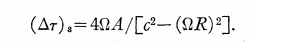The time interval of the rotating beam splitter is then computed to be: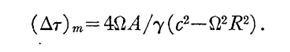Where g corresponds to the time dilation factor due to the rotational motion.  However, does the relativistic correction to the Sagnac equation actually make a difference in the actual result? When we consider the rotating Sagnac interferometer as a device experiencing time dilation, we need to consider first which parts are clocks and what the actual outcome time dilation will have on the measuring system, the detector. Let’s look at the Sagnac interferometer as three parts:

a)      A source such as a laser, which is a light clock subject to velocity time dilation

b)     An optical path composed of wavelengths of the emitted light

c)      A detector, which measures the phase difference between the wave-fronts of the two returning beams.

The source (for example a laser) is a true clock – lets assume that this clock experiences a time dilation proportional to

t’ = to/SQRT(1-WR^2/c^2)  = to * b .  then,

Dt = to * b - to   =  (2L- 2L/b)/C                                 (1)

This will inevitably mean that the rotating source must emit light at a reduced frequency, proportional to f’ = f * SQRT(1-WR^2/c^2). However, since C = f*l, the wavelengths in the optical path must expand due to the lower frequency. This means that there will be fewer wavelengths passing the detector per unit time.  The difference will be in the amount of time a given wave-front is now behind compared to the unaffected laser situation:

Dt = (2L- 2L/b)/C                                                    (2)

So the wave-fronts are behind by the amount Dt. But as we showed in 1, the time is also behind by the same amount Dt since the duration of each second is larger due to the clock frequency change. The result is that the two effects cancel out, and the same wave-front arrives at the detector at the same elapsed time regardless of the time dilation, from the standpoint of the observer on the disk. The relativistic correction would seem thereby to be unnecessary. As Post says, the result is too small to be experimentally distinguishable, and the common practice is to use a g factor = 1.

So this in and of itself does not contradict the notion that the measured speed of light by an observer on the disk is C +/- WR.

Post says himself that “the circulation times t1’ and t2’ in the rotating frame are obtained by integrating f’ from 0-2p and 0- -2p, respectively: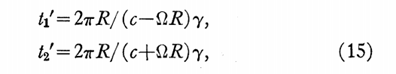And then it is easy to see that in the rotating frame:

Dt = L/(C+WR) – L/(C- WR) = 4AW/c^2                         (3)

Post quotes the method of Langevin from his 1921 and 1937 papers. In his 1921 paper, Langevin argued that because the Sagnac effect was first order, there could be no distinction between Newtonian or relativistic derivations and therefore the effect was in accordance with relativity theory. Dufour and Prunier took Langevin to task on this. Dufour and Prunier’s work is discussed by Kelly  in his book Challenging Modern Physics: Questioning Einstein's Relativity Theories”.    In their experiments they created Sagnac interferometers that were composites of moving and stationary paths, including stationary sources and stationary detectors. This was essentially to test if the relativistic approach could be distinguished from the classical approach. In particular, they focused on the mathematical treatment of Langevin, whereby the position placement of the observer on the disk is arbitrary. Using Langevin’s calculation:

Dt = L/C + 2WA/C^2                      (4)

They predicted that in a Sagnac interferometer where only part of the light path was rotating and the other part stationary, the classical equation would call for a different area A than the relativistic equation for calculating the result.  In all cases of this experimental test, the Sagnac effect was the same. This overturned Langevin’s analysis, and in 1937,  he had to revise his explanation, as pointed out by Kelly:

“In his final essay on the subject in 1937, Langevin proposed that the results published that year by Dufour and Prunier showed that one had to assume either (a) the light speed varied to c + wr in one direction and c – wr in the other direction, or (b) the time aboard the spinning apparatus had to change by a factor of +/-2wA/c2 in either direction. Indeed, Langevin went as far as to say that assuming (a), “we find, by a very simple and very general reasoning, the formula for the difference of the times of the path of the two light beams in the Sagnac experiment.” He also stated that “the paths are of unequal length because of the inequality of the speed of prorogation”.

The proposition (b) though is untenable because if this were true then when the light beam passed back to the moving detector, the local time from each direction would be out of synchronization, meaning that the clocks cannot be counting real time and that the effective time dilation is meaningless. This was also pointed out by Herbert Ives in his 1938 paper  criticizing Langevin. Ives says about the absurdity of Langevin’s proposition (b):

” There are of course not merely two clocks, but an infinity of clocks, where we include those that could be transported at finite speeds, and around other paths. As emphasized previously, the idea of “local time” is untenable, what we have are clock readings. Any number of clock readings at the same place are physically possible, depending on the behaviour and history of the  clocks used. More than one “time” at one place is a physical absurdity. “

The only explanation left, is Langevin’s proposition a) that the light speed varies by C+/-wr in one or the other direction around the disk, consistent with Dufour and Prunier’s experimental results.

References:

 Paul Langevin – (1937) On the Experiment of Sagnac. Compt. Rend. 205, 51.

[15} Paul Langevin – (1921) Compt. Rend. 173, 831.

 A. Dufour, F. Prunier,

 E. J. Post, Sagnac Effect, Review of Modern Physics, 39,2,